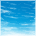# mx287 what would happen when sample voltage is much larger than VDDIO?

cancel
Showing results for
Did you mean:
SOLVED

## mx287 what would happen when sample voltage is much larger than VDDIO?

308 ViewsContributor III

1. As mentioned in the title, the iMX28_RM has a reference in chapter 38.2,  page 2662,

For input channels (other than BATT, 5V, or VDDIO) with signals larger than 1.85
V, the divide-by-two option should be set
set, the maximum input voltage is VDDIO – 50mv.

if our VDDIO is 3.3V,  does that mean the channels like lradc0 can't sample voltage which is larger than 3.3v minus 50mv?

2. If I configure the channel 0 as an analog input. So when one conversion is finished, the VALUE field in HW_LRADC_CH0 represents the actual voltage or I have to have it multiplied by voltage/per bit.

Thanks a lot.

Labels (2)

• ### Linux

1 Solution
206 ViewsNXP TechSupport

Q1. If our VDDIO is 3.3V, does that mean the channels like lradc0 can't sample voltage which is larger than 3.3v minus 50mv?

A1. It means that no voltage higher than 3.3v minus 50mv can be applied to the pin.

Q2. The VALUE field in HW_LRADC_CH0 represents the actual voltage or I have to have it multiplied by voltage/per bit.

A2. The measurement result is represented in the LRADC measurement units. Taking into the account the full input measurement range of 1.85V and the full range number of measurement units of 2^12 = 4096, the measurement unit value is 1.85V / 4096 = approx. 450uV. So, the actual measurement value in voltage units is the number in the VALUE field multiplied by 450uV.

Have a great day,
Artur

-----------------------------------------------------------------------------------------------------------------------
-----------------------------------------------------------------------------------------------------------------------

2 Replies
207 ViewsNXP TechSupport

Q1. If our VDDIO is 3.3V, does that mean the channels like lradc0 can't sample voltage which is larger than 3.3v minus 50mv?

A1. It means that no voltage higher than 3.3v minus 50mv can be applied to the pin.

Q2. The VALUE field in HW_LRADC_CH0 represents the actual voltage or I have to have it multiplied by voltage/per bit.

A2. The measurement result is represented in the LRADC measurement units. Taking into the account the full input measurement range of 1.85V and the full range number of measurement units of 2^12 = 4096, the measurement unit value is 1.85V / 4096 = approx. 450uV. So, the actual measurement value in voltage units is the number in the VALUE field multiplied by 450uV.

Have a great day,
Artur

-----------------------------------------------------------------------------------------------------------------------